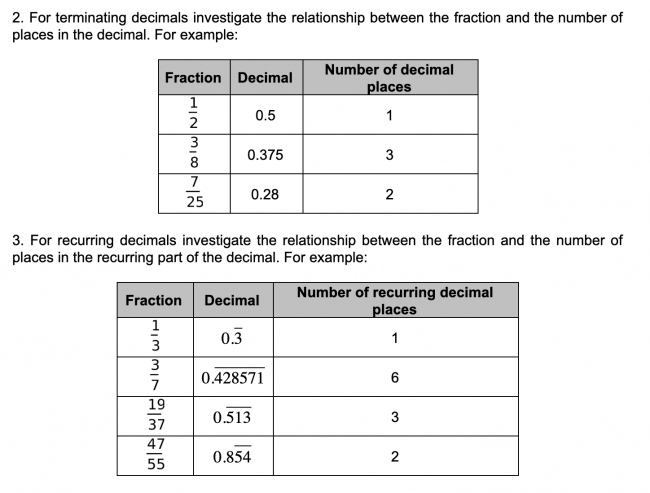# Fractions and Decimals

Preview:Students investigate for which fractions the decimal equivalent is terminating and for which it is recurring.

Project
Big Idea:
Grade 8: Number represents, describes, and compares the quantities of ratios, rates, and percents.
Grade 8: Computational fluency and flexibility extend to operations with fractions.
Curricular Competency:
Reasoning and analyzing: Use reasoning to explore and make connections
Understanding and solving: Develop, demonstrate, and apply mathematical understanding through play, inquiry, and problem solving
Communicating and representing: Communicate mathematical thinking in many ways
Communicating and representing: Use mathematical vocabulary and language to contribute to mathematical discussions
Communicating and representing: Explain and justify mathematical ideas and decisions